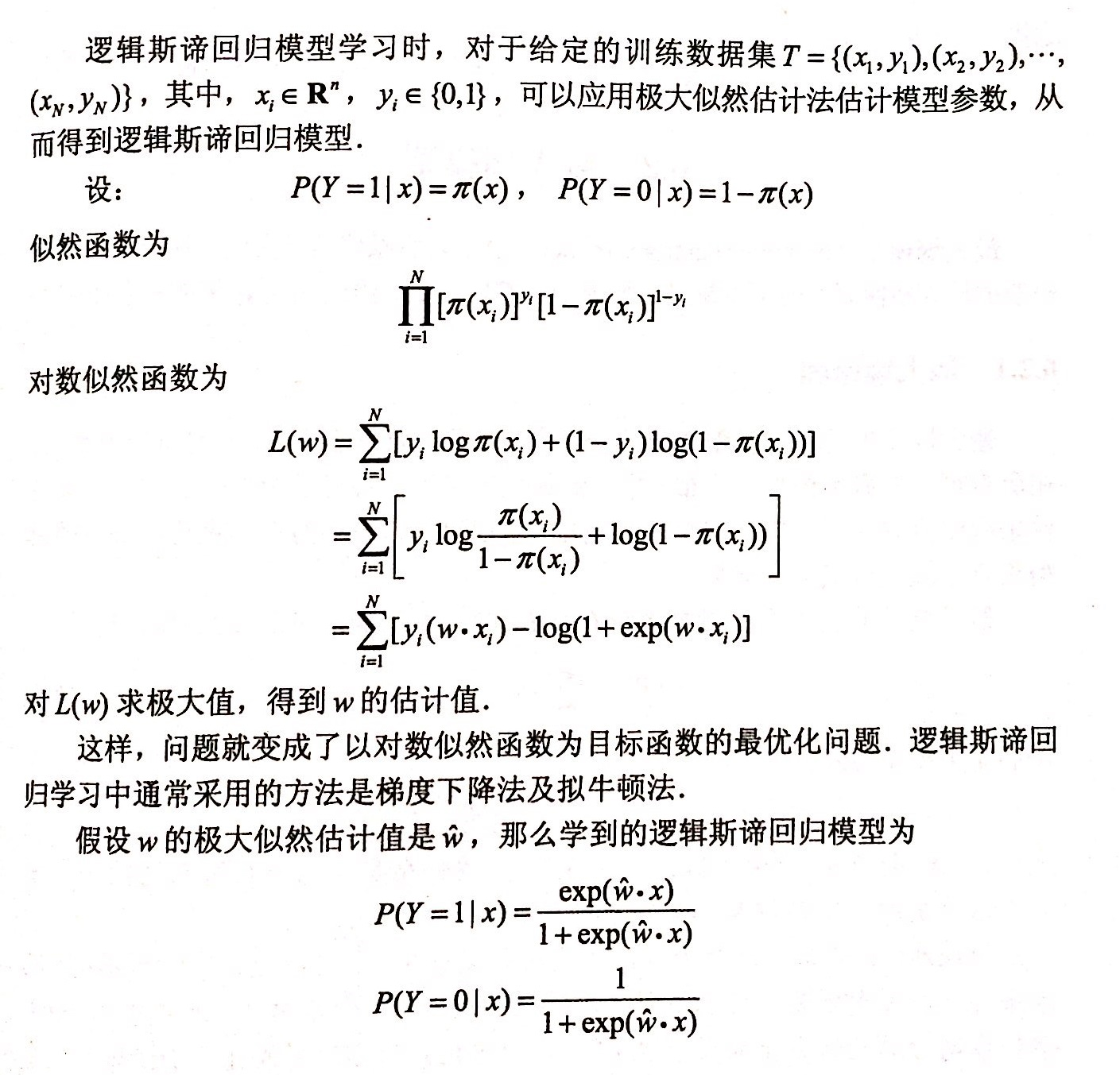# 【机器学习算法】：极大似然估计

#### 1.极大似然估计在什么情况下用

step1：已知一个模型（比如逻辑回归模型，线性回归模型）。
step2：给定已知的数据集D。
step3：用极大似然估计来估计step1中模型的参数。
step4：得到参数后，模型就完整啦~ 可以用完整的模型对未来的结果进行预测。

#### 2.为什么可以用极大似然估计来估计模型中的参数

• 似然函数定义：
假设数据集D服从一个参数为w的概率分布 f(x)=p(D=x|w) f ( x ) = p ( D = x | w ) $f(x) = p(D=x|w)$(含义就是知道w的条件下，数据集D取样本x的概率)， p(D|w) p ( D | w ) $p(D|w)$表示的是数据集D的联合概率分布，基于我们的独立性假设，该值为所有样本的概率密度函数 f(x) f ( x ) $f(x)$的乘积。我们将 p(D|w) p ( D | w ) $p(D|w)$称为参数w的似然函数。 log(p(D|w)) l o g ( p ( D | w ) ) $log(p(D|w))$为参数w的对数似然函数，记作 L(w|D) L ( w | D ) $L(w|D)$
Note:之所以取对数是因为后续会对似然函数求导，取对数后方便求导（对数运算将乘法变成了加法）

• 最大化似然函数估计参数
为什么我们可以通过最大化似然函数来估计模型参数呢。主要原因在于：样本数据集D是已经出现的状态，所以我们可以认为，D出现的概率比所有其他样本数据集D’出现的概率都要大，就是说 L(w|D)>L(w|D) L ( w | D ) > L ( w | D ′ ) $L(w|D) > L(w|D')$。所以说用极大似然估计得到一个参数w。

Wml=argmaxwlL(w|D)=argmaxwlog(p(D|w)) W m l = a r g m a x w l L ( w | D ) = a r g m a x w l o g ( p ( D | w ) ) $W_{ml}= argmax_{wl}L(w|D) = argmax_wlog(p(D|w))$

#### 3.以逻辑回归为例，极大似然法估计参数的过程11-02
11-303051
06-084938
10-233346
03-19261
06-051475
04-071227
04-131万+
08-203974
03-08761
08-071万+
10-08542
07-101万+
04-252264
05-23326
03-191万+
04-09190
09-061万+
12-09697

### “相关推荐”对你有帮助么？

•非常没帮助
•没帮助
•一般
•有帮助
•非常有帮助被折叠的  条评论 为什么被折叠?到【灌水乐园】发言yuanCruise

¥2 ¥4 ¥6 ¥10 ¥20余额支付 (余额：-- )扫码支付获取中扫码支付点击重新获取扫码支付1.余额是钱包充值的虚拟货币，按照1:1的比例进行支付金额的抵扣。
2.余额无法直接购买下载，可以购买VIP、C币套餐、付费专栏及课程。余额充值